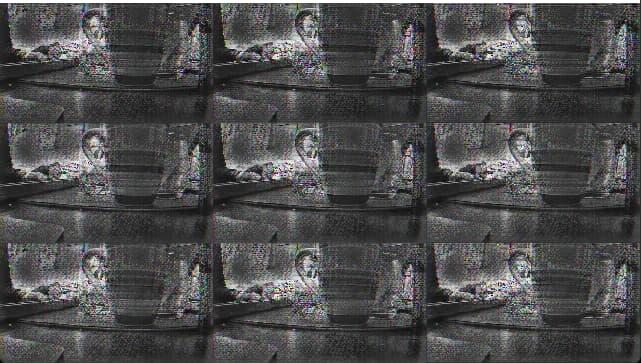# Torch::jit::load -> C++ vs Python

I used torch.jit.load with python and torch::jit::load with C++ (each 1.9.1 cu11.1).
The model that is transcripted is TransfomerNet as decribed in the following.
The results from python and C++ are differents as described by the images.

Python ResultC++ ResultBlockquote
class TransformerNetwork(nn.Module):

``````"""Feedforward Transformation Network without Tanh

reference: https://arxiv.org/abs/1603.08155

exact architecture: https://cs.stanford.edu/people/jcjohns/papers/fast-style/fast-style-supp.pdf

"""

def __init__(self, ConvLayer, ResidualLayer, DeconvLayer):

super(TransformerNetwork, self).__init__()

self.ConvBlock = nn.Sequential(

ConvLayer(3, 32, 9, 1),

nn.ReLU(),

ConvLayer(32, 64, 3, 2),

nn.ReLU(),

ConvLayer(64, 128, 3, 2),

nn.ReLU()

)

self.ResidualBlock = nn.Sequential(

ResidualLayer(128, 3),

ResidualLayer(128, 3),

ResidualLayer(128, 3),

ResidualLayer(128, 3),

ResidualLayer(128, 3)

)

self.DeconvBlock = nn.Sequential(

DeconvLayer(128, 64, 3, 2, 1),

nn.ReLU(),

DeconvLayer(64, 32, 3, 2, 1),

nn.ReLU(),

ConvLayer(32, 3, 9, 1, norm="None")

)

def forward(self, x):

x = self.ConvBlock(x)

x = self.ResidualBlock(x)

out = self.DeconvBlock(x)

return out
``````

class ConvLayer(nn.Module):

``````def __init__(self, in_channels, out_channels, kernel_size, stride, norm="instance"):

super(ConvLayer, self).__init__()

# Convolution Layer

self.conv_layer = nn.Conv2d(in_channels, out_channels, kernel_size, stride)

# Normalization Layers

self.norm_type = norm

if (norm=="instance"):

self.norm_layer = nn.InstanceNorm2d(out_channels, affine=True)

elif (norm=="batch"):

self.norm_layer = nn.BatchNorm2d(out_channels, affine=True)

def forward(self, x):

x = self.conv_layer(x)

if (self.norm_type=="None"):

out = x

else:

out = self.norm_layer(x)

return out
``````

class ResidualLayer(nn.Module):

``````"""

Deep Residual Learning for Image Recognition

https://arxiv.org/abs/1512.03385

"""

def __init__(self, channels=128, kernel_size=3):

super(ResidualLayer, self).__init__()

self.conv1 = ConvLayer(channels, channels, kernel_size, stride=1)

self.relu = nn.ReLU()

self.conv2 = ConvLayer(channels, channels, kernel_size, stride=1)

def forward(self, x):

identity = x                     # preserve residual

out = self.relu(self.conv1(x))   # 1st conv layer + activation

out = self.conv2(out)            # 2nd conv layer

out = out + identity             # add residual

return out
``````

class DeconvLayer(nn.Module):

``````def __init__(self, in_channels, out_channels, kernel_size, stride, output_padding, norm="instance"):

super(DeconvLayer, self).__init__()

# Transposed Convolution

# Normalization Layers

self.norm_type = norm

if (norm=="instance"):

self.norm_layer = nn.InstanceNorm2d(out_channels, affine=True)

elif (norm=="batch"):

self.norm_layer = nn.BatchNorm2d(out_channels, affine=True)

def forward(self, x):

x = self.conv_transpose(x)

if (self.norm_type=="None"):

out = x

else:

out = self.norm_layer(x)

return out
``````

Blockquote

The C++ result looks interleaved, which is often caused by a wrongly used `view` or `reshape` where a `permute` would be needed.
I don’t see any data processing code posted, but I would guess that you are using `PIL` in Python to load the image (channels-first by default) and OpenCV in C++ (channels-last). In C++ you might have been running into a shape mismatch error and tried to use `view/reshape` to create an input tensor of `[batch_size, channels, height, width]`. If so, use `permute` to permute the axes to create a channels-first tensor.

Unfortunately, the input input tensor is `[batch_size, channels, height, width]` and I used `permute` to achieve it.
I used the same pipeline with other C++ network and it’s worked well. That’s why I asked this question. I can’t understand where the issue comes from. I supposed it may come from the transcription from the python network to c++ network but it works when I used torch.jit.load.
For the transcription, I used only trace. The `.graph` and the .`code` do not help me well.
It may come from `dtype` issue. I’ll investigate on this.

That’s weird, as I’m pretty sure you are displaying an interleaved image.
You can easily reproduce it by loading the first (properly displayed) image and by running this code:

``````import PIL
import numpy as np
import matplotlib.pyplot as plt

img = PIL.Image.open("./discuss01.jpeg")
arr = np.array(img)
shape = arr.shape
print(shape)
plt.imshow(arr)

arr_interleaved = arr.transpose(2, 0, 1).reshape(shape)
plt.imshow(arr_interleaved)
``````

which will result exactly in the interleaved second output you’ve posted.

Thanks, I run your code and it seems to be like I have. So my pipeline has an issue :
` tensor = torch::from_blob(open_cv.data, { 1, height, width, channels = 3 }, at::kByte).to(at::kFloat).div(255).permute({ 0, 3, 1, 2 }))` gives a Tensor of dimension `{1, 3, height, width}`.

Then I do :
`model.forward({ tensor }).toTensor().to(at::kByte).squeeze().permute({ 1,2,0 })` to have a tensor of dimension `{height, width, 3}` for OpenCV displaying.
When I do not do `model.forward` the result is normal.

With your help I investigate in the right direction. The problem is solved when I use `contiguous()` on the net result. I need to understand more the methods that modifies the memory and the others that is just a “view” of it.
Thanks1 Like